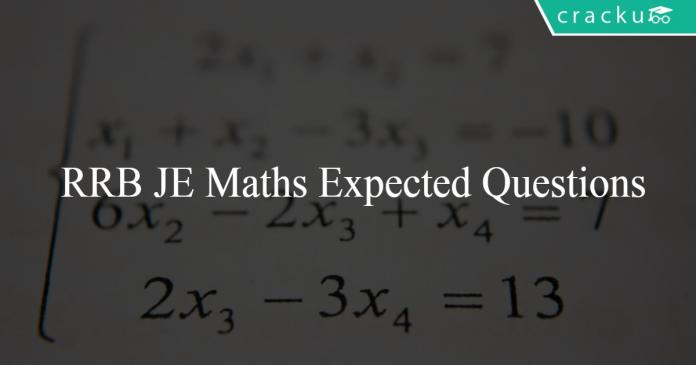# RRB JE Maths Expected Questions 2019

0
613# RRB JE Maths Expected Questions 2019

Download Top 10 RRB JE Expected Maths Questions and Answers PDF. RRB JE Maths Questions based on asked questions in previous exam papers very important for the Railway JE exam.

Question 1: Find the value of $\dfrac{1}{sin A} – \dfrac{sin A}{cos^2 A}$.

a) 0

b) 1

c) 2cot 2A sec A

d) 2 tan 2A sec A

Question 2: If a = 6, b = 2 and c = -8, then what is the value of $a^3 + b^3 + c^3$?

a) 0

b) -2146

c) -286

d) -288

Question 3: The present ages of Amit and Balu are in the ratio of 4 : 3. After 10 years, their ages will be in the ratio 6 : 5. Find the present age of Balu.

a) 18 years

b) 15 years

c) 21 years

d) 24 years

Question 4: 2.1221-3.1331+4.1441 = ?

a) 9.3993

b) 3.1331

c) 6.2662

d) none of these

Question 5: Study the following information and answer the question below:
Loss in millions for companies A,B,C and D in the years 2017,2018 and 2019 is given in the line chart.

Which company has consistent increase in loss from years 2017 to 2019 ?

a) B

b) A

c) D

d) C

Question 6: What is the HCF of 1/15,4/3 and 7/6 ?

a) 1/30

b) 4/30

c) 7/30

d) 28/30

Question 7: There are 83 people standing in the queue to buy movie tickets. Alok is 37th from the start while Sachin is 68th from the start. How many people are standing between Alok and Sachin?

a) 28

b) 30

c) 29

d) 31

Question 8: Find the sum of the following series upto 10 terms:
1*3 + 2*4 + 3*5 + 4*6 + …..

a) 505

b) 495

c) 515

d) 485

Question 9: There are 83 people standing in the queue to buy movie tickets. Alok is 37th from the start while Sachin is 68th from the start. How many people are standing between Alok and Sachin?

a) 28

b) 30

c) 29

d) 31

Question 10: 22 boys are standing in front of Kunal in a Queue 12 boys are standing to the back of Rohit in the same queue. If total number of boys is 30 then the number of boys standing in between Kunal and Rohit is

a) 3

b) 4

c) 6

d) 5

$1/sinA – SinA/Cos^2A$
= $(cos^2A-sin^2A)/(sinA.cos^2A)$
= $2(Cos2A)/(sin2A.CosA)$
= $2Cot2A.secA$
So the answer is option A

Given, a = 6, b = 2, c = -8
Here, a+b+c = 0.
We know that if a+b+c = 0, then $a^3+b^3+c^3 = 3abc$
Therefore, $a^3 + b^3 + c^3 = 3 \times 6 \times 2 \times (-8) = -288$

Let the present ages of Amit and Balu be 4x years and 3x years respectively.
After 10 years, their ages will be 4x+10 years and 3x+10 years respectively.
Given, $\dfrac{4x+10}{3x+10} = \dfrac{6}{5}$
⇒ 20x+50 = 18x+60
⇒ 2x = 10
⇒ x = 5.
Hence, The present age of Balu is 3x = 3*5 = 15 years.

2.1221-3.1331+4.1441 = -1.0110+4.1441 = 3.1331

So the answer is option B.

From the line chart we can say that D is the only company in which loss has increased each year from 2017 to 2019 i.e 1,3 and 4 in each years respectively

HCF of fractions=HCF of numerators/LCM of denominators
HCF of 1,4,7=1
LCM of 15,3,6 is 30

Alok is 37th from the start while Sachin is 68th from start
Hence the number of people between Sachin and Alok will be 68 – 37 – 1 = 30
Hence, option B is the right choice.

The given terms can be generalized as $(n-1)(n+1)$ starting from $n=2$.
$(n+1)(n-1) = n^2 -1$
$n$ will vary from $2$ to $11$.

Therefore, sum of the series = $(1^2+2^2 + 3^2 + 4^2 +…..11^2)-1 – (1+1+…10 \text{terms})$.
= $\frac{n(n+1)(2n+1)}{6} -10$
=$\frac{11*12*23}{6} – 11$
=$506 -11$
=$495$.

Therefore, option B is the right answer.